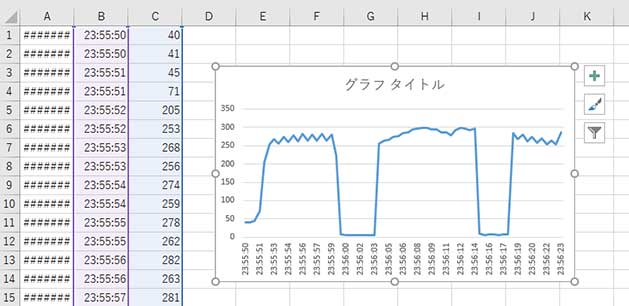## Overview

With GR-PEACH, save the data in CSV format on the SD Card and display a graph in excel file. This includes applied usage such as reading of real-time-clock (RTC) or the value on analog.

## Preparation

You will need a GR-PEACH board, USB cable (Micro B type), Micro SD card, illuminance sensor (NJL7502L), and 10kΩ of resistance. You can try half of this project even you don't have an illuminance sensor.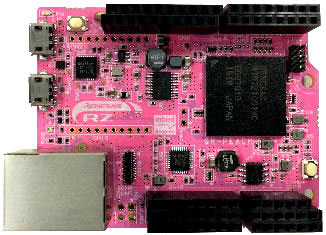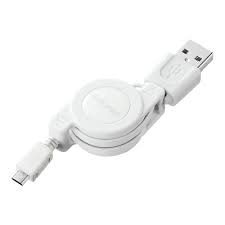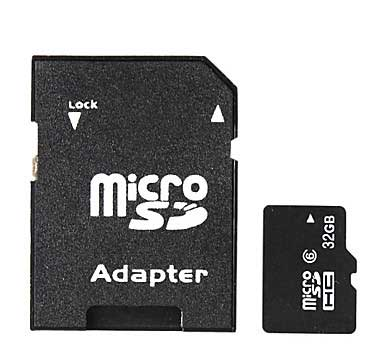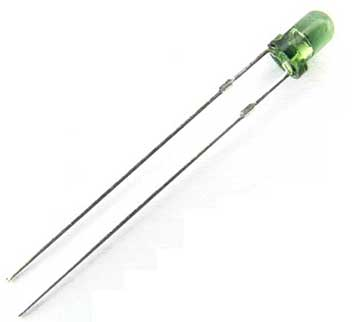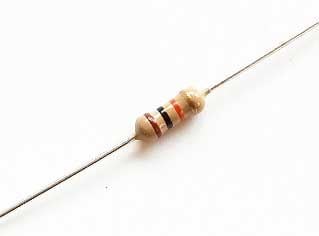## Working Out the Clock

Execute the sample program below to have the green LED flicker every 1 second.

``````
#include <arduino.h>
#include <RTC.h>

RTC rtc;
static int year, mon, day, hour, min, sec, week;

void setup() {
pinMode(PIN_LED_GREEN, OUTPUT);
digitalWrite(PIN_LED_GREEN, LOW); // LED OFF
Serial.begin(9600);

year = 2016;
mon  = 7;
day  = 23;
hour = 13;
min  = 20;
sec  = 50;
week = RTC_WEEK_SATURDAY;

rtc.begin();
rtc.setDateTime(year, mon, day, hour, min, sec, week);

}

void loop() {
static int sec_old = sec;
rtc.getDateTime(year, mon, day, hour, min, sec, week);

if((sec - sec_old) % 60){
delay(5);
digitalWrite(PIN_LED_GREEN, LOW);
Serial.print(year); Serial.print("/");
Serial.print(mon);  Serial.print("/");
Serial.print(day);  Serial.print(" ");
Serial.print(hour); Serial.print(":");
Serial.print(min);  Serial.print(":");
Serial.print(sec);
Serial.println();
sec_old = sec;
}
}

``````

A serial monitor such as Tera Term or CoolTerm shows time every 1 second. This is used for the timestamp of data storage on an SD card or time –axis as data logger.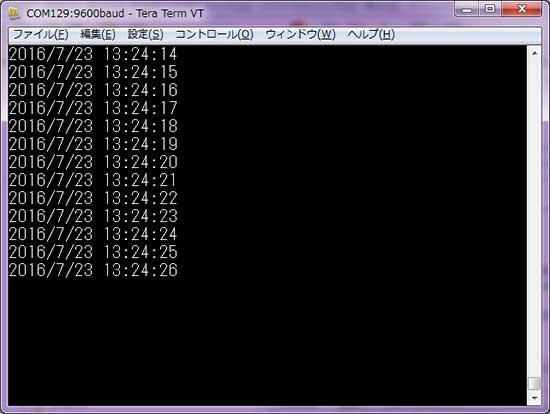## Working Out the Graph After Data Storage on an SD Card

Next, here is a sample program to save the data on an SD card. Insert a  Micro SD into the socket on the back of GR-PEACH to execute a sketch. When writing to the SD card has successfully completed, the green LED will flicker. When you fail to access the  SD Card, the red LED flashes, please make sure the Micro SD is inserted correctly.

``````
#include <arduino.h>
#include <RTC.h>
#include <SD.h>

RTC rtc;
static int year, mon, day, hour, min, sec, week;
void sd_timestamp(uint16_t* date, uint16_t* time);

void setup() {
pinMode(PIN_LED_GREEN, OUTPUT);
pinMode(PIN_LED_RED, OUTPUT);
digitalWrite(PIN_LED_GREEN, LOW);
digitalWrite(PIN_LED_RED, LOW);

Serial.begin(9600);
if (!SD.begin()) {
digitalWrite(PIN_LED_RED, HIGH); // error
while(1);
}

SdFile::dateTimeCallback( &sd_timestamp );

year = 2016;
mon  = 7;
day  = 23;
hour = 13;
min  = 20;
sec  = 50;
week = RTC_WEEK_SATURDAY;

rtc.begin();
rtc.setDateTime(year, mon, day, hour, min, sec, week);

}

void loop() {
static int deg = 0;
File file = SD.open("data.csv", FILE_WRITE);
if (file) {
digitalWrite(PIN_LED_GREEN, HIGH);
rtc.getDateTime(year, mon, day, hour, min, sec, week);
// date
file.print(year);   file.print("/");
file.print(mon);    file.print("/");
file.print(day);
file.print(",");
// time
file.print(hour);   file.print(":");
file.print(min);    file.print(":");
file.print(sec);
file.print(",");
// data
file.print(sin(deg * PI / 180.0));
file.print(",");
file.print(cos(deg * PI / 180.0));
file.println();
file.close();
deg+=5;
digitalWrite(PIN_LED_GREEN, LOW);
}

delay(500);
}

void sd_timestamp(uint16_t* date, uint16_t* time)
{
*date = FAT_DATE(year, mon, day);
*time = FAT_TIME(hour, min, sec);
}
``````

Remove the SD card when the timing is appropriate, check the status with the PC to see if the file was created on the SD card.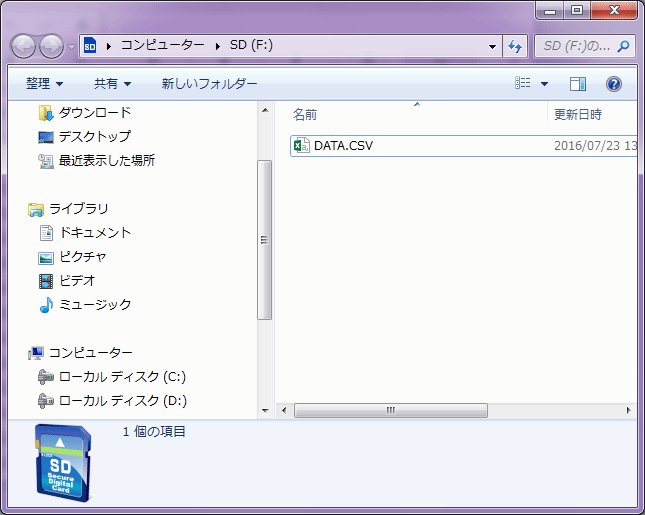Open the DATA.CSV file with a spreadsheet program such as Microsoft Excel.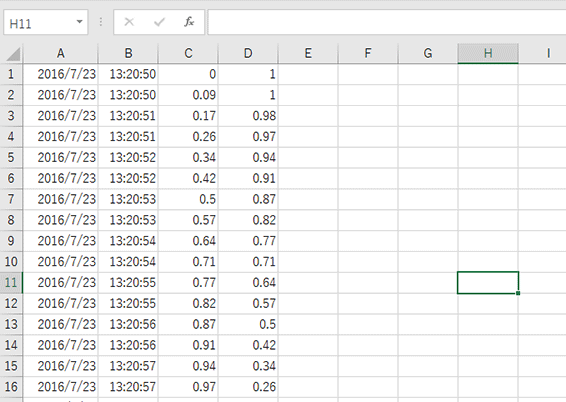You can see the wave form of Sin and Cos in the graph.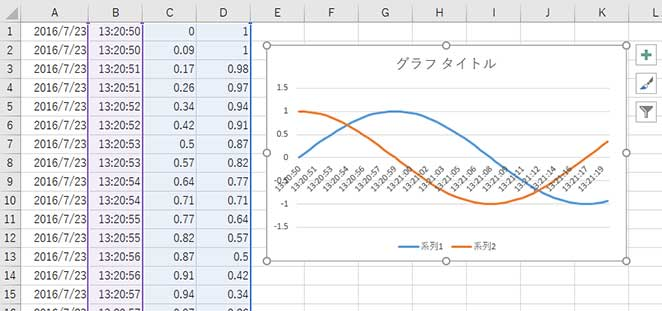## Working Out the Logger on the Illuminance Sensor

Finally, we introduce the sample program for data storage on an SD card, measuring with the illuminance sensor. The different from the program above is reading the analog value of the A0 pin with analogRead (A0) and saving to the SD card. Distinguishing from the above program, once writing on an SD card is successfully complete, the blue LED flickers. Let's connect the illuminance sensor and resistance to execute the sample program.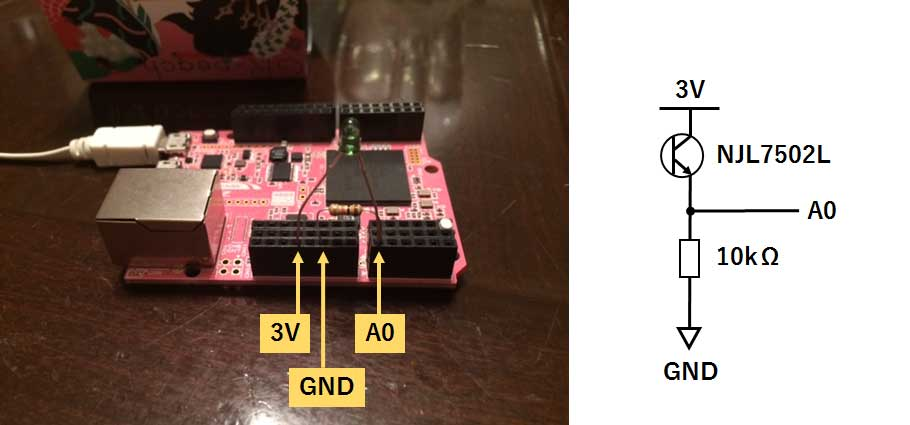``````
#include <arduino.h>
#include <RTC.h>
#include <SD.h>

RTC rtc;
static int year, mon, day, hour, min, sec, week;
void sd_timestamp(uint16_t* date, uint16_t* time);

void setup() {
pinMode(PIN_LED_BLUE, OUTPUT);
pinMode(PIN_LED_RED, OUTPUT);
digitalWrite(PIN_LED_BLUE, LOW);
digitalWrite(PIN_LED_RED, LOW);

Serial.begin(9600);
if (!SD.begin()) {
digitalWrite(PIN_LED_RED, HIGH); // error
while(1);
}

SdFile::dateTimeCallback( &sd_timestamp );

year = 2016;
mon  = 7;
day  = 28;
hour = 23;
min  = 55;
sec  = 50;
week = RTC_WEEK_THURSDAY;

rtc.begin();
rtc.setDateTime(year, mon, day, hour, min, sec, week);

}

void loop() {
File file = SD.open("data.csv", FILE_WRITE);
if (file) {
digitalWrite(PIN_LED_BLUE, HIGH);
rtc.getDateTime(year, mon, day, hour, min, sec, week);
// date
file.print(year);   file.print("/");
file.print(mon);    file.print("/");
file.print(day);
file.print(",");
// time
file.print(hour);   file.print(":");
file.print(min);    file.print(":");
file.print(sec);
file.print(",");
// data
file.println();
file.close();
digitalWrite(PIN_LED_BLUE, LOW);
}

delay(500);
}

void sd_timestamp(uint16_t* date, uint16_t* time)
{
*date = FAT_DATE(year, mon, day);
*time = FAT_TIME(hour, min, sec);
}
``````

According to variation of the illuminance, you can see the numerical value change in the graph. For example, a low value shows the low illuminance. The unit of the value of this sample program is not LUX. To know the value of illuminance, calculation is required, considering the spec of the illuminance sensor and changing the resistance level depends on the surrounding environment.